# How to Invoke Shared Formulas in a Canvas PowerApp

### Colin Maitland, 30 October 2019

In this article, I demonstrate how to invoke a shared formula from events and controls in a Canvas PowerApp without the need to duplicate the formula many times.

The following image shows a simple calculator created as a Canvas PowerApp. This calculator has various buttons, labels and associated formulas.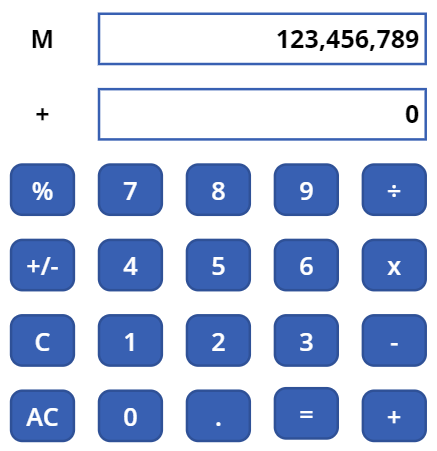Firstly, the formula used to initialise this dynamically sizable calculator is invoked from two locations. From the OnVisible event of the screen on which the calculator is placed, and from the OnSelect event of a User clickable Initialise button, named “CalculatorButtonInitialise”, on the same screen.

Here is the formula associated with the Initialise button. This formula also invokes a formula associated with the OnSelect event of the AC (All Clear) button on the calculator. This is done using the Select function; i.e. Select(CalculatorButtonAllClear). “CalculatorButtonAllClear” is the name of the AC button on the calculator.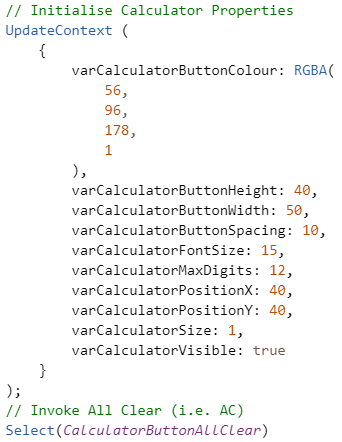Here is the formula associated with the OnVisible event of the screen. This formula uses the Select function to invoke the formula associated with the OnSelect event of the Initialise button; i.e. Select(CalculatorButtonInitialise). “CalculatorButtonInitialise” is the name of the Initialise button.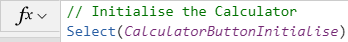Here is the formula associated with the OnSelect event of the AC (All Clear) button on the calculator. The purpose of this formula is to clear the current operator, value and total.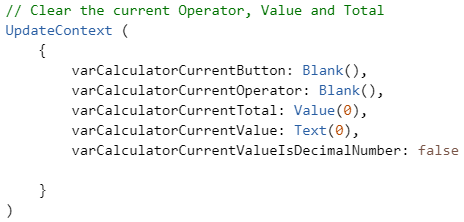This approach demonstrated here removes the need to duplicate the same formulas when invoked from different events and controls.

I will now demonstrate this approach for a longer and more complex shared formula invoked from many controls.

The same formula needs to be invoked when the user presses any of the 0 to 9 or the decimal point buttons on the calculator. The formula consists of common logic divided into three parts. Here is the third part of this formula that shows updating the current displayed value being entered by the user; e.g. updating the current value from 0 to 1.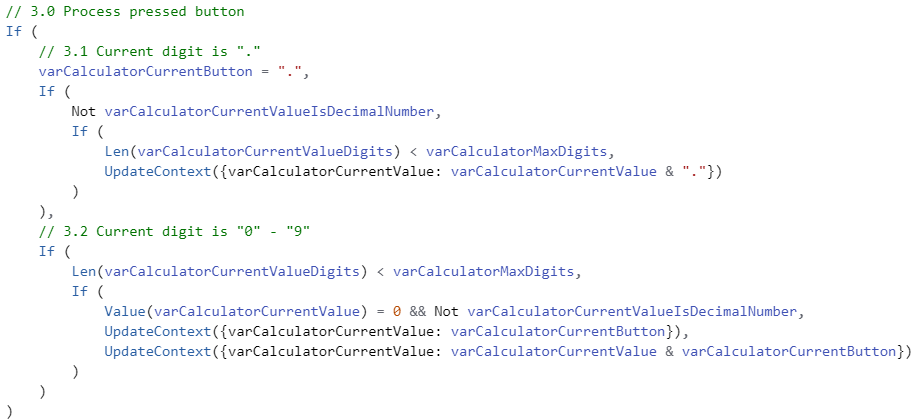To avoid having a copy of this entire formula associated with each of the number and the decimal point buttons on the calculator, I have associated it with the OnSelect event of a hidden button named “CalculatorFunctionDigitOnSelect” on the same screen.

Each of the number and the decimal point buttons on the calculator has the following two-line formula associated with their OnSelect events.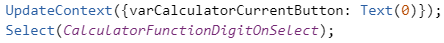The first line of these formulas set a context variable named “varCalculatorCurrentButton” to the corresponding button; either 0 to 9 or decimal point. This variable is used as a parameter by the formula associated with the OnSelect event of the hidden “CalculatorFunctionDigitOnSelect” button.

The second line of these formulas uses the Select function to invoke the OnSelect event of the hidden “CalculatorFunctionDigitOnSelect” button. Invoking this event results in the shared formula being executed.

This approach has removed the need to duplicate the shared formula many times, once for each button.

I have also used this approach with the operator buttons on the calculator; i.e. ÷, x, -, +, % and =. I associated the shared function for these buttons with the OnSelect event of a hidden button named “CalculatorFunctionOperatorOnSelect”.

Each of the operator buttons on the calculator has the following two line-formula associated with their OnSelect events.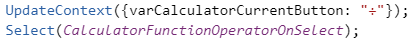As with the previous example, the first line of these formulas sets a context variable named “varCalculatorCurrentButton” to the corresponding button; either ÷, x, -, +, % or =. This variable is used as a parameter by the formula associated with the OnSelect event of the hidden “CalculatorFunctionOperatorOnSelect” button.

The second line of these formulas uses the Select function to invoke the OnSelect event of the hidden “CalculatorFunctionOperatorOnSelect” button. Invoking this event results in the shared formula being executed.

Here is part of this shared formula showing branched logic that performs division or multiplication by a percentage; e.g. 100 x 10%.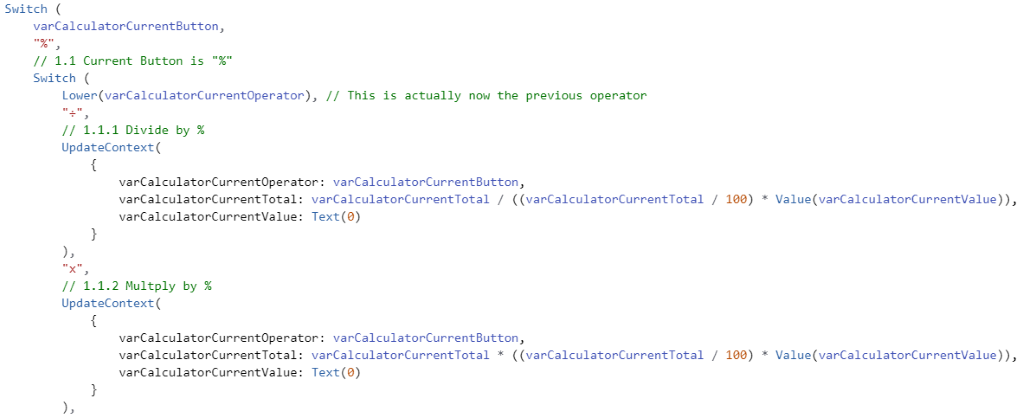In conclusion, it is possible to write, maintain and invoke shared formulas in a Canvas PowerApp using this approach. This approach simplifies the writing and testing of such formulas and reduces the occurrence of bugs and inconsistencies being introduced into these formulas because they only exist in one place rather than in multiple places.